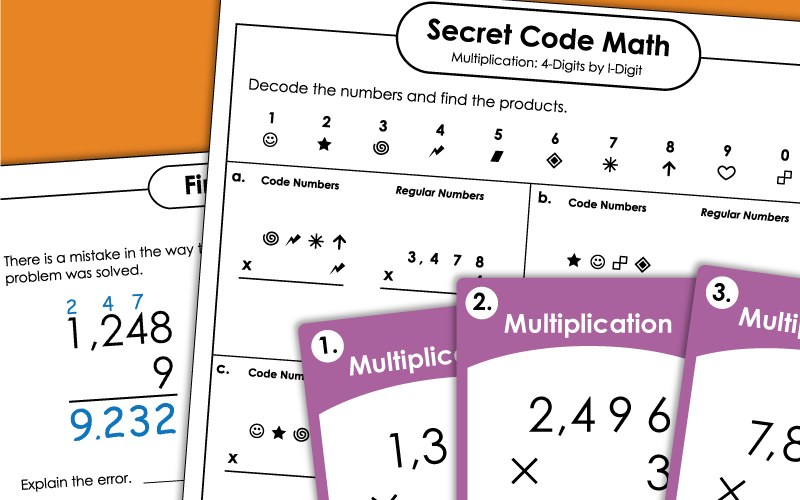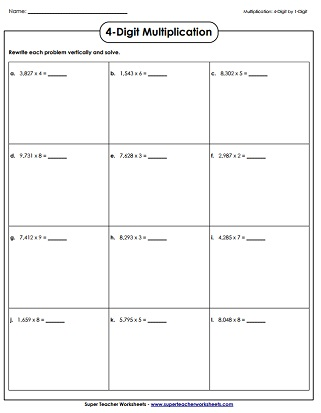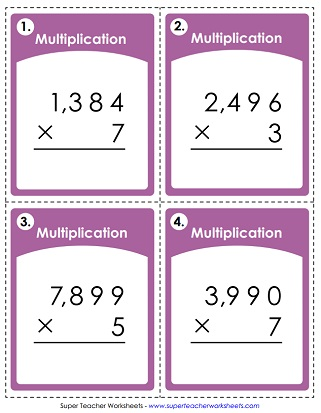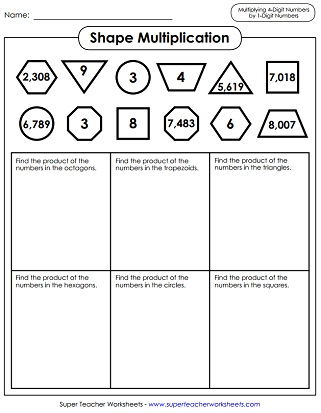# Multiplication Printables (4-Digits Times 1-Digit)

The multiplication worksheets below require students to find the products of 4-digit numbers and single-digit numbers.## 4-Digit Times 1-DigitWorksheets

Solve these 4-digit numbers by 1-digit problems. Includes 10 vertical problems and 2 word problems.
This worksheet has a series of 4-digit by 1-digit horizontal multiplication problems. Students rewrite the math problems vertically and solve. (example: 6,741 x 4)
Solve a variety of multiplication problems, such as horizontal and vertical equations, word problems, and solving for a variable.
If you looks closely at these completed multiplication problems, you'll notice that each one has an error. Student are to find and explain the errors, then re-solve.
At he top of this page, students will see shapes with numbers in them. They multiply similar shapes together. For example: Find the product of the numbers in the hexagons.
Kids love these math code activities. First they use the key to decode the mystery symbols. Then they multiply the numbers together.

Multiplication Worksheet Generator

Create your own multiplication worksheets! You choose the number of digits in the factors. Also tell if you would like to include money or word problems.

This set of 30 task cards has 4-digit by 1-digit problems for students to solve. Use these for classroom scavenger hunts, small group instruction, or peer study sessions.

## Multiplication Patterns

Place value patterns in multiplication; Learn to use mental math to multiply large numbers that end in zero (example: 400 x 6)
More Multi-Digit Multiplication

## Worksheet ImagesMy Account
Site Information